0
In cart:
0 items\$0.00
Search and Filter Items
Search Products
OR Browse Departments

Filter Results

# Peppers and other Miscellaneous Vegetables

Product Size Price Quantity

L
Per Pound
\$11.49
lb

## English Cucumbers

CFL
Each (price approx.)
\$2.07You are ordering units, but this item is sold by the pound. The price per pound is \$4.49 and the average weight for one item is 0.46 lbs., therefore, the approximate price for one item is \$2.07. Please order the number of items you would like to receive. Your final price will be calculated based on the total weight of the actual items fulfilled.

7 oz
\$13.99

CFLOG
0.75 oz
\$7.99

## Organic Aloe Leaf

OG
Per Pound
\$2.29
lb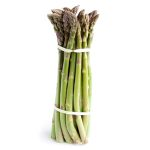## Organic Asparagus

CFLOG
Each (price approx.)
\$11.99You are ordering units, but this item is sold by the pound. The price per pound is \$7.99 and the average weight for one item is 1.50 lbs., therefore, the approximate price for one item is \$11.99. Please order the number of items you would like to receive. Your final price will be calculated based on the total weight of the actual items fulfilled.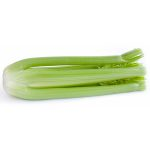## Organic Celery

OG
Per Pound
\$1.49
lb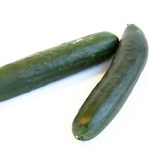## Organic Cucumber

OG
Each (price approx.)
\$2.49You are ordering units, but this item is sold by the pound. The price per pound is \$2.49 and the average weight for one item is 1.00 lbs., therefore, the approximate price for one item is \$2.49. Please order the number of items you would like to receive. Your final price will be calculated based on the total weight of the actual items fulfilled.

## Organic Fava Beans

OG
Per Pound
\$2.99
lb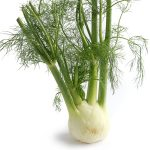## Organic Fennel

OG
Each (price approx.)
\$2.79You are ordering units, but this item is sold by the pound. The price per pound is \$2.79 and the average weight for one item is 1.00 lbs., therefore, the approximate price for one item is \$2.79. Please order the number of items you would like to receive. Your final price will be calculated based on the total weight of the actual items fulfilled.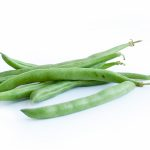## Organic Green Beans

OG
Per Pound
\$2.99
lb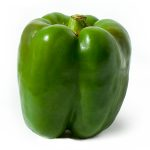## Organic Green Bell Pepper

OG
Each (price approx.)
\$1.40You are ordering units, but this item is sold by the pound. The price per pound is \$3.49 and the average weight for one item is 0.40 lbs., therefore, the approximate price for one item is \$1.40. Please order the number of items you would like to receive. Your final price will be calculated based on the total weight of the actual items fulfilled.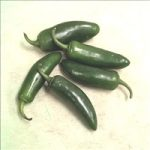OG
Per Pound
\$3.99
lb

OG
16 oz
\$5.49

OG
16 oz
\$3.49

## Organic Orange Bell Peppers

OG
Each (price approx.)
\$2.32You are ordering units, but this item is sold by the pound. The price per pound is \$5.79 and the average weight for one item is 0.40 lbs., therefore, the approximate price for one item is \$2.32. Please order the number of items you would like to receive. Your final price will be calculated based on the total weight of the actual items fulfilled.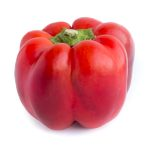## Organic Red Bell Peppers

OG
Each (price approx.)
\$2.00You are ordering units, but this item is sold by the pound. The price per pound is \$4.99 and the average weight for one item is 0.40 lbs., therefore, the approximate price for one item is \$2.00. Please order the number of items you would like to receive. Your final price will be calculated based on the total weight of the actual items fulfilled.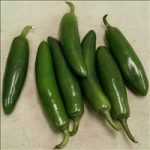## Organic Serrano Pepper

OG
Per Pound
\$3.49
lb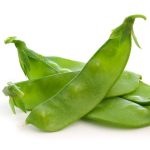## Organic Snow Peas

OG
Per Pound
\$5.49
lb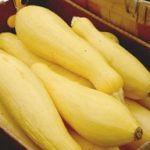## Organic Squash Yellow

OG
Each (price approx.)
\$1.29You are ordering units, but this item is sold by the pound. The price per pound is \$2.99 and the average weight for one item is 0.43 lbs., therefore, the approximate price for one item is \$1.29. Please order the number of items you would like to receive. Your final price will be calculated based on the total weight of the actual items fulfilled.

## Organic Sugarsnap Peas

OG
Per Pound
\$3.29
lb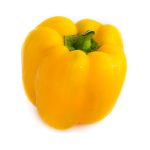## Organic Yellow Bell Pepper

OG
Each (price approx.)
\$2.20You are ordering units, but this item is sold by the pound. The price per pound is \$5.49 and the average weight for one item is 0.40 lbs., therefore, the approximate price for one item is \$2.20. Please order the number of items you would like to receive. Your final price will be calculated based on the total weight of the actual items fulfilled.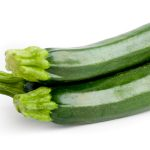## Organic Zucchini

OG
Each (price approx.)
\$1.02You are ordering units, but this item is sold by the pound. The price per pound is \$3.29 and the average weight for one item is 0.31 lbs., therefore, the approximate price for one item is \$1.02. Please order the number of items you would like to receive. Your final price will be calculated based on the total weight of the actual items fulfilled.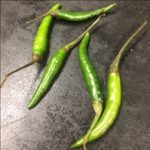Per Pound
\$12.49
lb